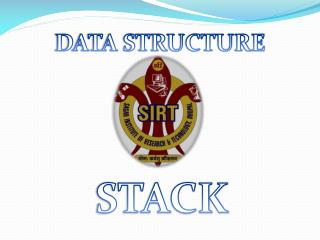DownloadDownload PresentationSTACK

# STACK

Télécharger la présentation## STACK

- - - - - - - - - - - - - - - - - - - - - - - - - - - E N D - - - - - - - - - - - - - - - - - - - - - - - - - - -
##### Presentation Transcript

1. DATA STRUCTURE STACK

2. Stack • A stack is a list of element in which an element may be inserted or deleted only at one end, called the top of the stack. This means in particular ,that elements are removed from a stack in the reverse order of thet in which they were inserted into the stack. • Stack is also called last-in-first –out (LIFO)type of list .

3. Example of stack • Stack of book • Stack of pennies

4. Two basic operation • PUSH is the term used to insert an element into a stack • POP is the term used to delete an element from a stack

5. Diagram of push operation top top F E A B C D top E A B C D A B C D top Push( F) Push (E)

6. Diagram of POP operation A B C D A B C A B top top top POP( C) POP (D)

7. Implementation of stack • Static implementation of stack • Dynamic implementation of stack

8. Static implementation of stack • Static implementation of stack uses of array to create stack. • Therefore a stack can be declared as a structure containing two fields . Ex: #define MAXSIZE 100 Strcut stack{int top; int element[MAXSIZE] }; Struct stack s;

9. Dynamic implementation of stack • Dynamic implementation is also called linked list representation and uses pointer to implement the stack type of data structure • Ex: Strcut node{int info; int node *next; }; Struct node *s;